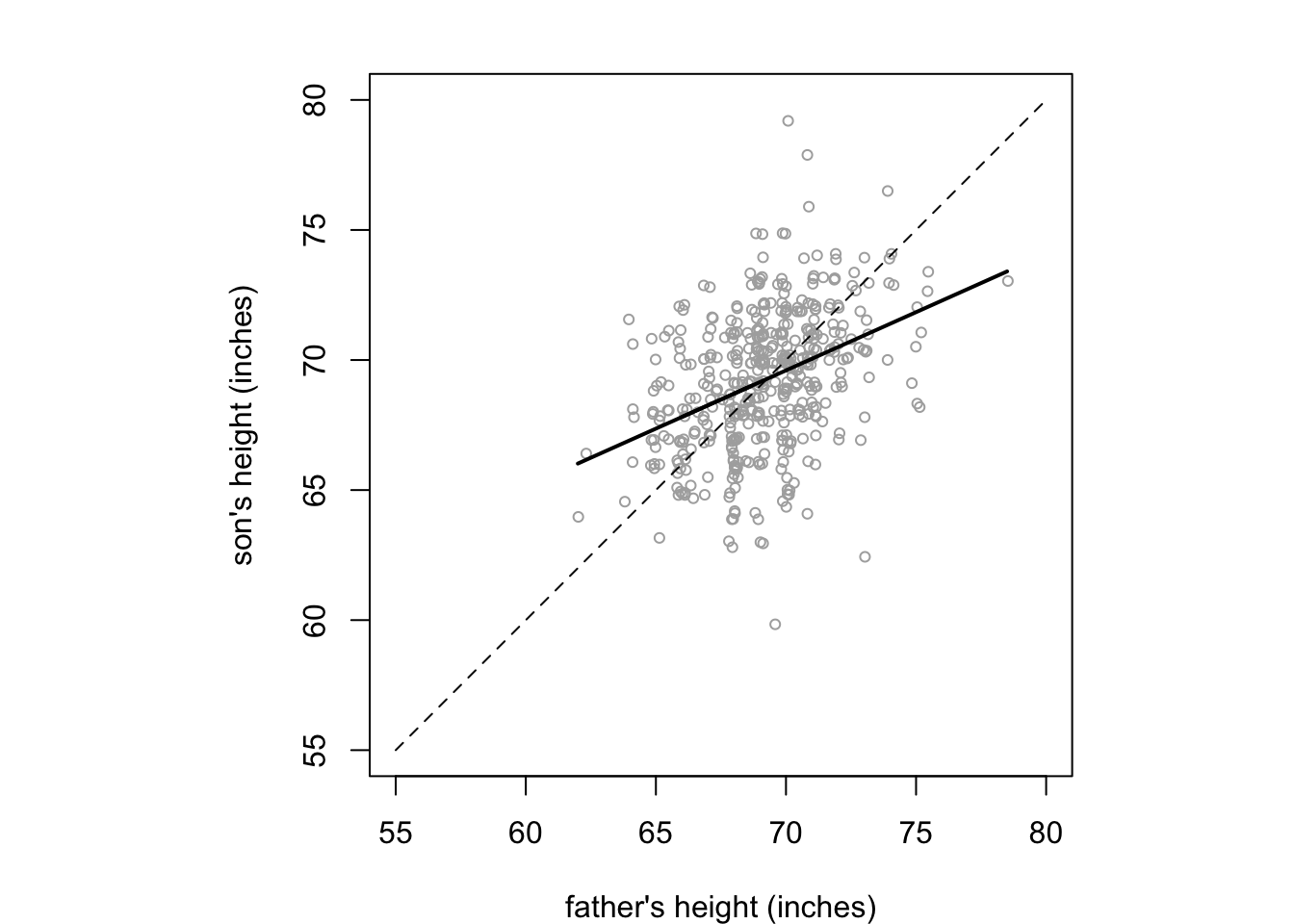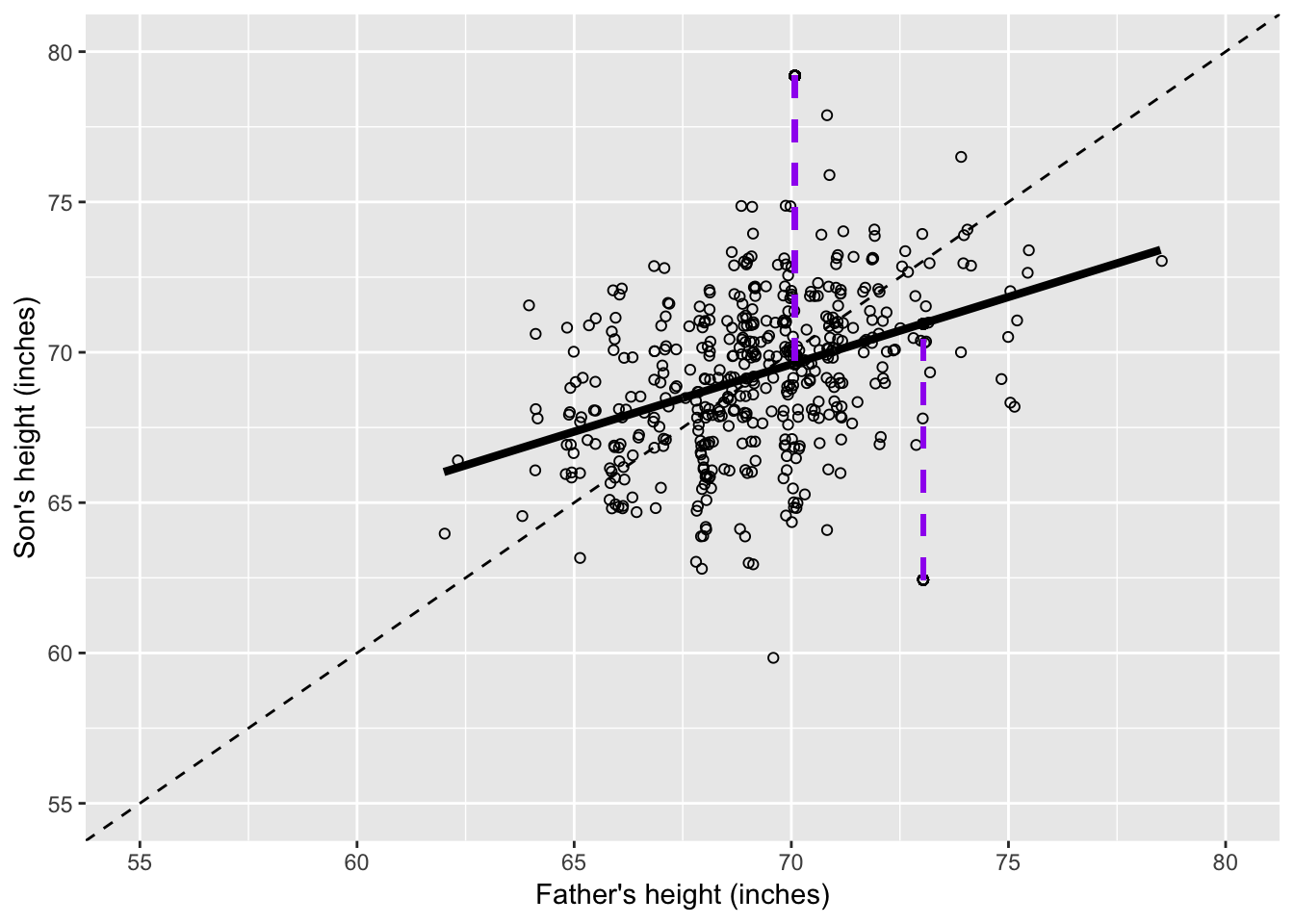Data from 1991-1995 are contained in 05-1-galton-x.csv, Although the book says the data is from HistData: Data Sets from the History of Statistics and Data Visualization, 2018, I have actually used this version of Galton’s Height Data

attach(galton) #uncomment if/while necessary

summary(galton)
##      Family        Father          Mother      Gender      Height
##  185    : 15   Min.   :62.00   Min.   :58.00   F:433   Min.   :56.00
##  166    : 11   1st Qu.:68.00   1st Qu.:63.00   M:465   1st Qu.:64.00
##  66     : 11   Median :69.00   Median :64.00           Median :66.50
##  130    : 10   Mean   :69.23   Mean   :64.08           Mean   :66.76
##  136    : 10   3rd Qu.:71.00   3rd Qu.:65.50           3rd Qu.:69.70
##  140    : 10   Max.   :78.50   Max.   :70.50           Max.   :79.00
##  (Other):831
##       Kids
##  Min.   : 1.000
##  1st Qu.: 4.000
##  Median : 6.000
##  Mean   : 6.136
##  3rd Qu.: 8.000
##  Max.   :15.000
##
# summary statistics
# need means for unique fathers and mothers - identify first mention of each family
Unique.Fathers=numeric()
Unique.Mothers=numeric()
nunique=1 # number of unique families
Unique.Fathers = Father
Unique.Mothers = Mother
for(i in 2:length(Family))
{
if(Family[i] != Family[i-1]){
nunique=nunique+1
Unique.Fathers[nunique]=Father[i]
Unique.Mothers[nunique]=Mother[i]
}
}

length(Unique.Fathers)
##  197
summary(Unique.Fathers)
##    Min. 1st Qu.  Median    Mean 3rd Qu.    Max.
##   62.00   68.00   69.50   69.35   71.00   78.50
sd(Unique.Fathers)
##  2.622034
length(Unique.Mothers)
##  197
summary(Unique.Mothers)
##    Min. 1st Qu.  Median    Mean 3rd Qu.    Max.
##   58.00   62.70   64.00   63.98   65.50   70.50
sd(Unique.Mothers)
##  2.355607
Son = Height[Gender=="M"]
length(Son)
##  465
summary(Son)
##    Min. 1st Qu.  Median    Mean 3rd Qu.    Max.
##   60.00   67.50   69.20   69.23   71.00   79.00
sd(Son)
##  2.631594
Daughter = Height[Gender=="F"]
length(Daughter)
##  433
summary(Daughter)
##    Min. 1st Qu.  Median    Mean 3rd Qu.    Max.
##   56.00   62.50   64.00   64.11   65.50   70.50
sd(Daughter)
##  2.37032

Figure 5.1 (page 124) Linear regression of sons’ on fathers’ heights

# Heights of fathers of sons
FatherS = Father[Gender=="M"]

fit <- lm(Son ~ FatherS) # linear regression data in fit
Predicted <- predict(fit)   # Get the predicted values
summary(fit)
##
## Call:
## lm(formula = Son ~ FatherS)
##
## Residuals:
##     Min      1Q  Median      3Q     Max
## -9.3774 -1.4968  0.0181  1.6375  9.3987
##
## Coefficients:
##             Estimate Std. Error t value Pr(>|t|)
## (Intercept) 38.25891    3.38663   11.30   <2e-16 ***
## FatherS      0.44775    0.04894    9.15   <2e-16 ***
## ---
## Signif. codes:  0 '***' 0.001 '**' 0.01 '*' 0.05 '.' 0.1 ' ' 1
##
## Residual standard error: 2.424 on 463 degrees of freedom
## Multiple R-squared:  0.1531, Adjusted R-squared:  0.1513
## F-statistic: 83.72 on 1 and 463 DF,  p-value: < 2.2e-16
FatherS.j <- jitter(FatherS, factor=5)
Son.j <- jitter(Son, factor=5)

xlims=ylims=c(55,80)
par(mfrow=c(1,1), mar=c(4,4,2,0), pty="s")  # square plot

plot(FatherS.j, Son.j, xlim=xlims,ylim=ylims,cex=0.7,
xlab="father's height (inches)",ylab="son's height (inches)" , col="gray68")
lines(c(xlims,xlims),c(xlims,xlims),lty=2 )
lines(Predicted~FatherS,lwd=2)Now in ggplot

library(ggplot2)
# create new data frame with exact and jittered, and predcted values

Males = cbind.data.frame(FatherS,FatherS.j,Son,Son.j,Predicted)

p <- ggplot(Males, aes(x=FatherS, y=Son)) # initial plot object
p <- p + geom_point(x=FatherS.j,y=Son.j,shape= 1) # defines scatter type plot
p <- p + labs(x="Father's height (inches)", y= "Son's height (inches)") # adds x and y axis labels
p <- p + theme(legend.position="none")#, legend.box = "horizontal") # removes the legend
p <- p + expand_limits(x = c(55,80),y = c(55,80)) # expand the axis limits
p <- p + geom_line(aes(FatherS,Predicted),size=1.5) # add previously fitted linear regression line

p <- p + geom_abline(slope=1, linetype="dashed") # line to represent equality between son and father height

# select single data points by CSV datarow numbers
pointA=c(137)
pointB=c(28)

# plot residual line and end points for selectedpointA
p <- p + geom_point(aes(x=FatherS.j[pointA], y = Predicted[pointA]), shape = 1)
p <- p + geom_point(aes(x=FatherS.j[pointA], y = Son.j[pointA]), shape = 1)
p <- p + geom_segment(linetype="dashed", size=1, colour="purple",aes(x=FatherS.j[pointA],y=Son.j[pointA],xend = FatherS.j[pointA], yend = Predicted[pointA])) #p <- p + p

# plot residual line and end points for pointB
p <- p + geom_point(aes(x=FatherS.j[pointB], y = Predicted[pointB]), shape = 1)
p <- p + geom_point(aes(x=FatherS.j[pointB], y = Son.j[pointB]), shape = 1)
p <- p + geom_segment(linetype="dashed", size=1, colour="purple",aes(x=FatherS.j[pointB],y=Son.j[pointB],xend = FatherS.j[pointB], yend = Predicted[pointB]))

p #displays the resultFigure 5.1 Scatter of heights of 465 fathers and sons from Galton’s data (many fathers are repeated since they have multiple sons). A jitter has been added to separate the points, and the diagonal dashed line represents exact equality between son and father’s heights. The solid line is the standard ‘best-fit’ line. Each point gives rise to a ‘residual’ (dashed line), which is the size of the error were we to use the line to predict a son’s height from his father’s.